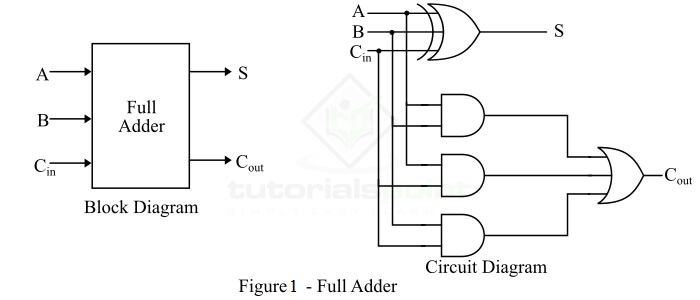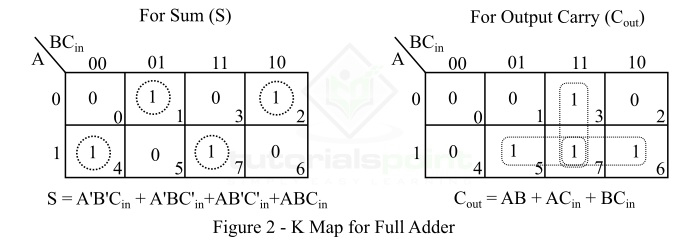# Full Adder in Digital Electronics

Addition is one of the most basic operations performed by different electronic devices like computers, calculators, etc. The electronic circuit that performs the addition of two or more numbers, more specifically binary numbers, is called as adder. As we know, the logic circuits use binary number system to perform the operations, hence the adder is referred to as binary adder.

Depending on the number of binary digits that a circuit can add, adders (or binary adders) are of two types −

In this tutorial, we will discuss the Full Adder, its definition, circuit diagram, truth table, k-map, characteristic equations, and applications.

## What is a Full Adder?

A combinational logic circuit that can add two binary digits (bits) and a carry bit, and produces a sum bit and a carry bit as output is known as a full-adder.

In other words, a combinational circuit which is designed to add three binary digits and produces two outputs (sum and carry) is known as a full adder. Thus, a full adder circuit adds three binary digits, where two are the inputs and one is the carry forwarded from the previous addition. The block diagram and circuit diagram of the full adder are shown in Figure-1.Hence, the circuit of the full adder consists of one EX-OR gate, three AND gates and one OR gate, which are connected together as shown in the full adder circuit in Figure-1.

Full adder takes three inputs namely A, B, and Cin. Where, A and B are the two binary digits, and Cin is the carry bit from the previous stage of binary addition. The sum output of the full adder is obtained by XORing the bits A, B, and Cin. While the carry output bit (Cout) is obtained using AND and OR operations.

## Truth Table of Full Adder

Truth table is one that indicates the relationship between input and output variables of a logic circuit and explains the operation of the logic circuit. The following is the truth table of the full-adder circuit −

Inputs Outputs
A B Cin S (Sum) Cout (Carry)
0 0 0 0 0
0 0 1 1 0
0 1 0 1 0
0 1 1 0 1
1 0 0 1 0
1 0 1 0 1
1 1 0 0 1
1 1 1 1 1

Hence, from the truth table, it is clear that the sum output of the full adder is equal to 1 when only 1 input is equal to 1 or when all the inputs are equal to 1. While the carry output has a carry of 1 if two or three inputs are equal to 1.

K-Map (Karnaugh Map) is a tool for simplifying binary complex Boolean algebraic expressions. The K-Map for full adder is shown in Figure-2.### Characteristic Equations of Full Adder

The characteristic equations of the full adder, i.e. equations of sum (S) and carry output (Cout) are obtained according to the rules of binary addition. These equations are given below −

The sum (S) of the full-adder is the XOR of A, B, and Cin. Therefore,

$$\mathrm{Sum,\, S=A\oplus B\oplus C_{in}=A'B'C_{in}+A'BC'_{in}+AB'C'_{in}+ABC_{in} }$$

The carry (C) of the half-adder is the AND of A and B. Therefore,

$$\mathrm{Carry,\, C=AB+AC_{in}+BC_{in}}$$

• Full adder provides facility to add the carry from the previous stage.

• The power consumed by the full adder is relatively less as compared to half adder.

• Full adder can be easily converted into a half subtractor just by adding a NOT gate in the circuit.

• Full adder is one of the essential part of critic digital circuits like multiplexers.

• Full adder performs operation at higher speed.

The following are the important applications of full adder −

• Full adders are used in ALUs (arithmetic logic units) of CPUs of computers.

• Full adders are used in calculators.

• Full adders also help in carrying out multiplication of binary numbers.

• Full adders are also used to realize critic digital circuits like multiplexers.